# 0.展示PTA总分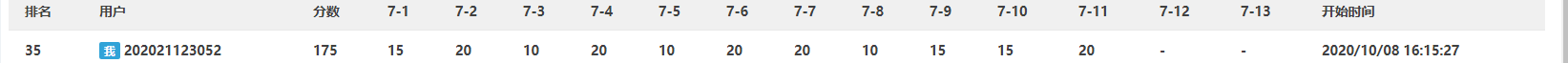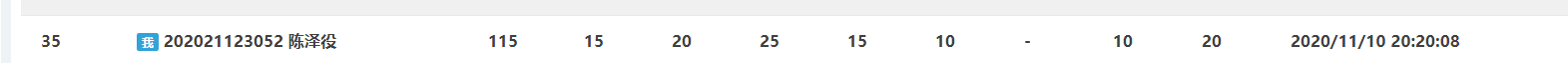# 1.本章学习总结

## 1.1 for循环语法

for（语句1；语句2；语句3）
{
循环体；
}


## 1.2 while、do while循环语法

while(条件)
{
循环体;
}

• while(1)为死循环,可能造成运算超时。

## 1.3 跳出循环相关语句break\continue

### 1.3.1 break语句

#include <stdio.h>
int main()
{
int i = 1, sum = 0;
while (1)
{
sum =sum+i;
i++;
if (i > 100)
break;//用break打断，并退出循环。
}
printf("%d", sum);
return 0;
}


### 1.3.2 continue语句

unsigned char i, j=50;
for(i=0;i<100;i++)
{
if(i>j) continue;//满足条件则不用执行下面语句，直接进入下一次循环。
j=i;
}


## 1.4 循环嵌套

• for循环、while循环、do-while循环可以嵌套使用，即外循环和内循环。
• 案例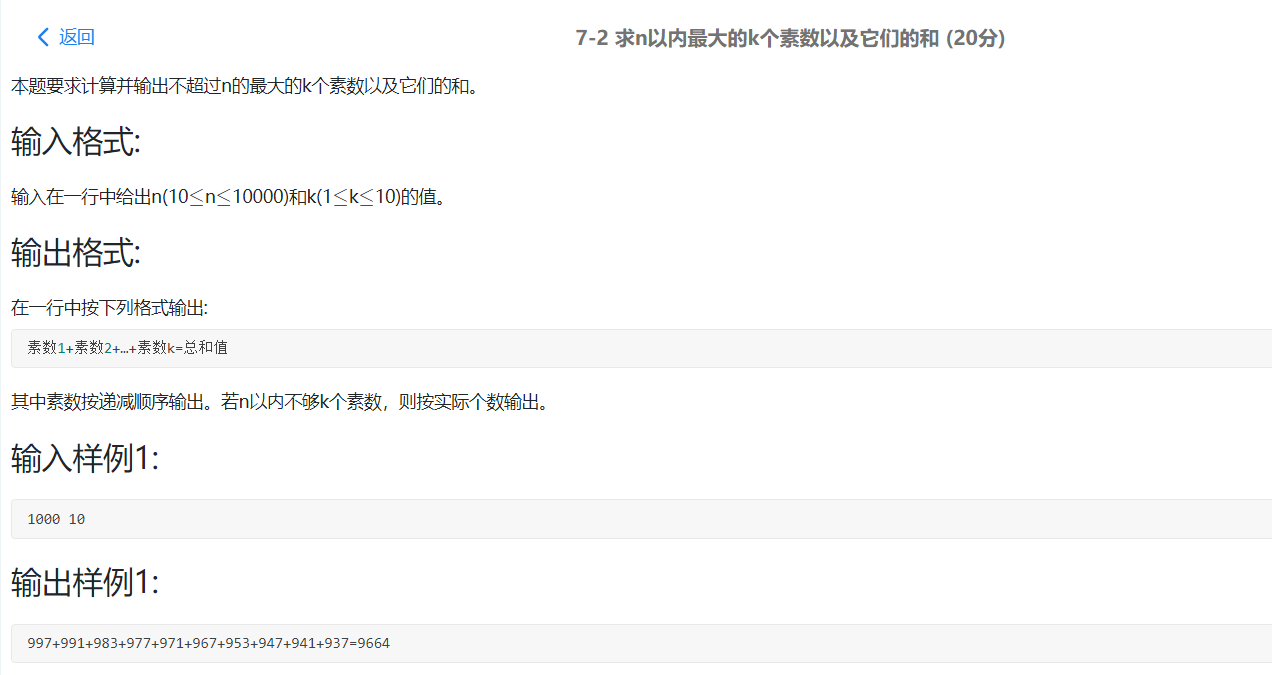#include<stdio.h>
#include<math.h>
int main()
{
int n,k,i,j,m,h,sum=0,count=0;
10<=n<=10000,1<=k<=10;
m=sqart(i);
scanf("%d %d",&n,&k);
for(i=n;i>=10;i--)
for(h=2;h<=m;h++){
if(i%h==0)
break;
if(h>m){
count++;
sum+=i;
}
if(count=k+1)
break;
}
}
printf("%d",sum);
return 0;
}


# 2.编程技巧总结

1.数列求和

• 主义：求和初始值一定要定义sum=0。
2.字符型
• 技巧：
①转成数字
ch = '1'; number = ch - '0';//则得出数字一


②字母大小写

ch = 'b'; ch = ch + 'A' - 'a';//则实现大小写转换


③打印图形

# 3.PTA实验作业

## 3.1 数列求和问题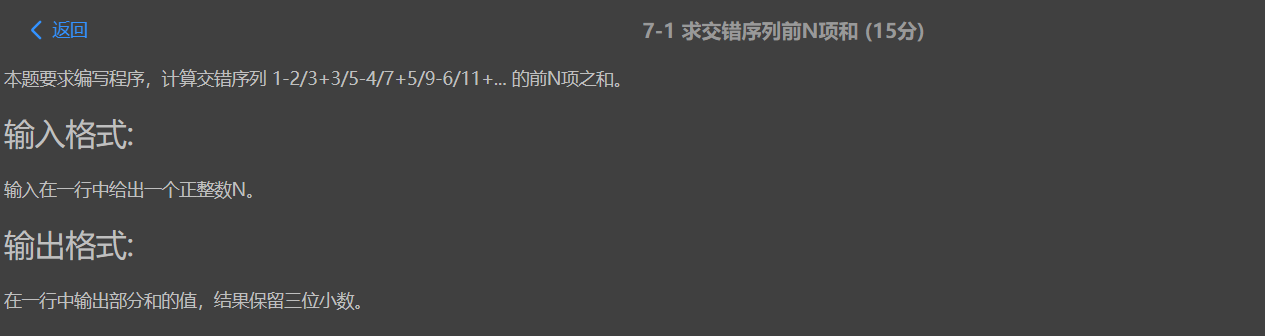### 3.1.1 流程图或伪代码介绍思路

• 定义sum为整型数0，通过for循环写出每项的通项公式，然后累加。

### 3.1.2 代码截图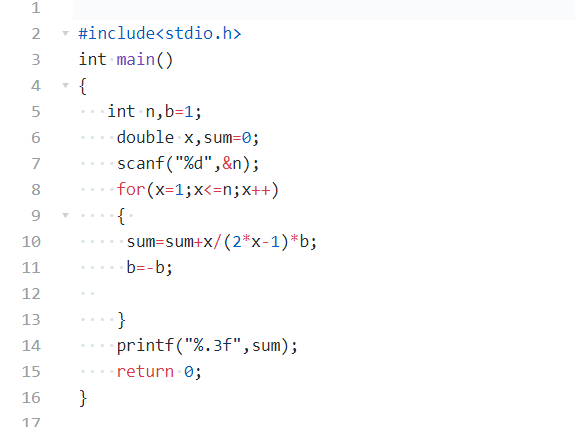### 3.1.3 PTA提交列表及说明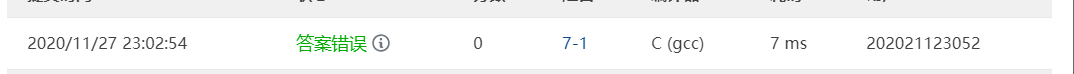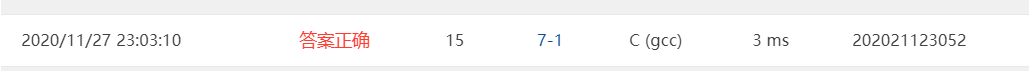• 输出数据的时候没有限制小数点位数，导致答案错误。

## 3.2 图形打印问题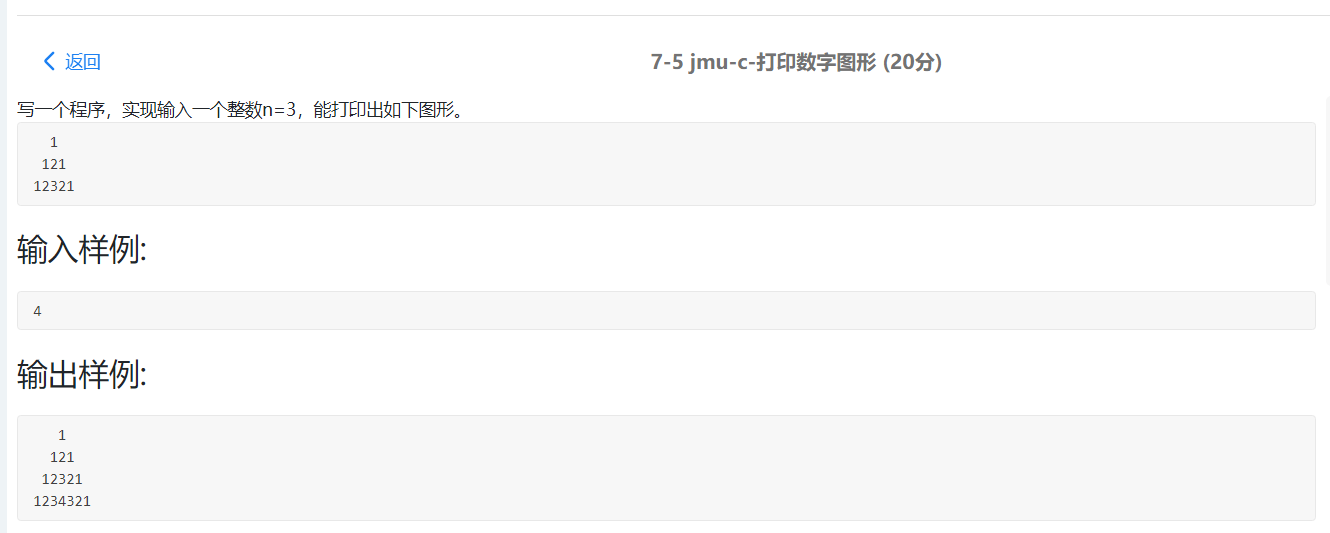### 3.2.1 数据处理

• 根据对应的循环然后打出相应空格，在按顺序打出数字。

### 3.2.2 代码截图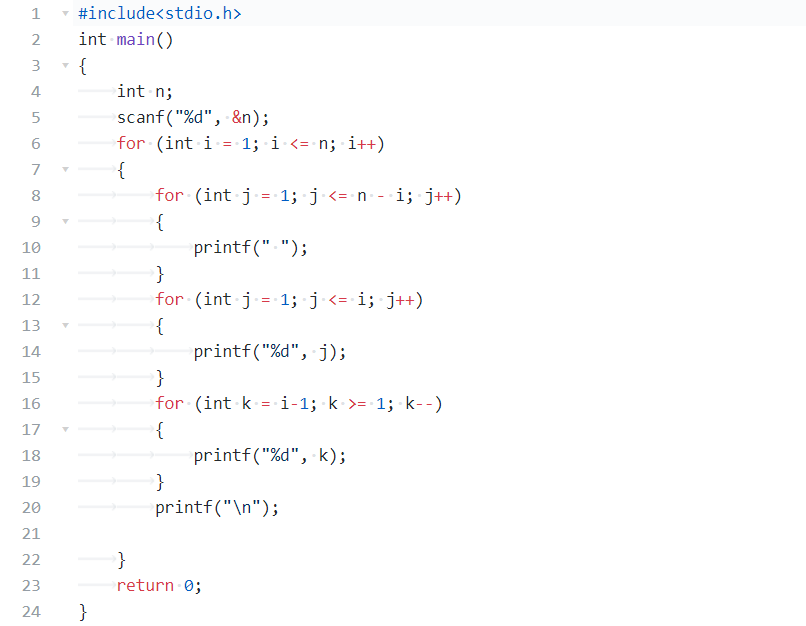### 3.3 自选一题，介绍printf调试如何检查错误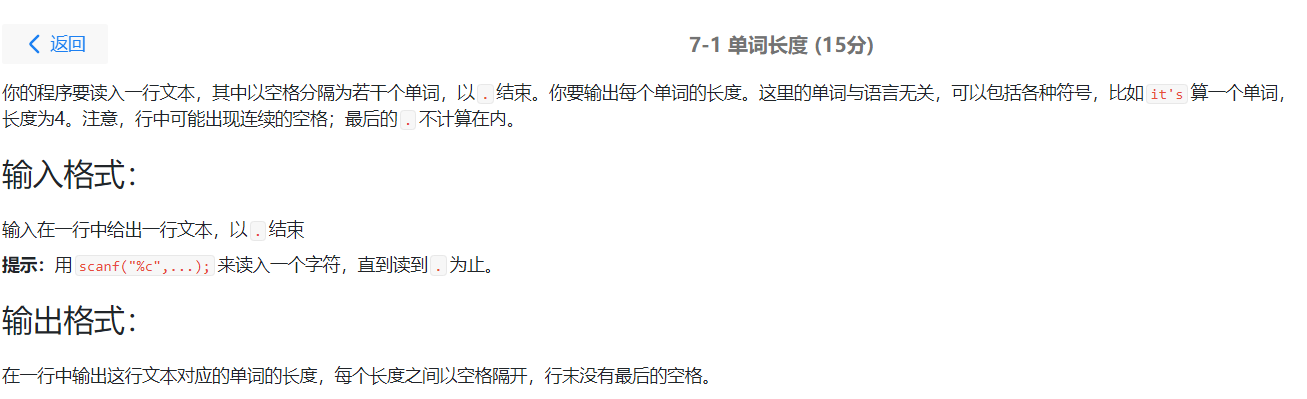### 3.3.1 单步调试截图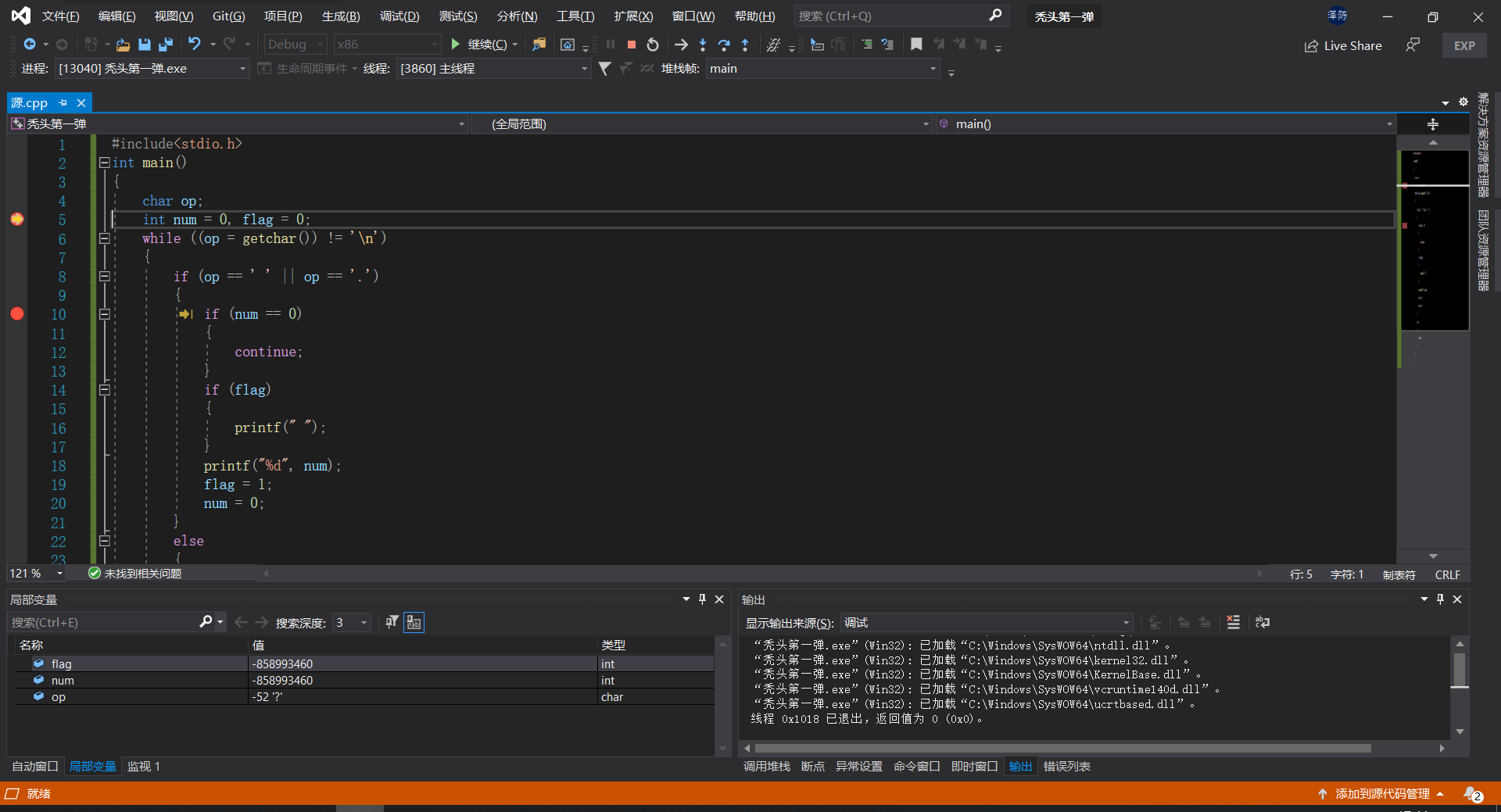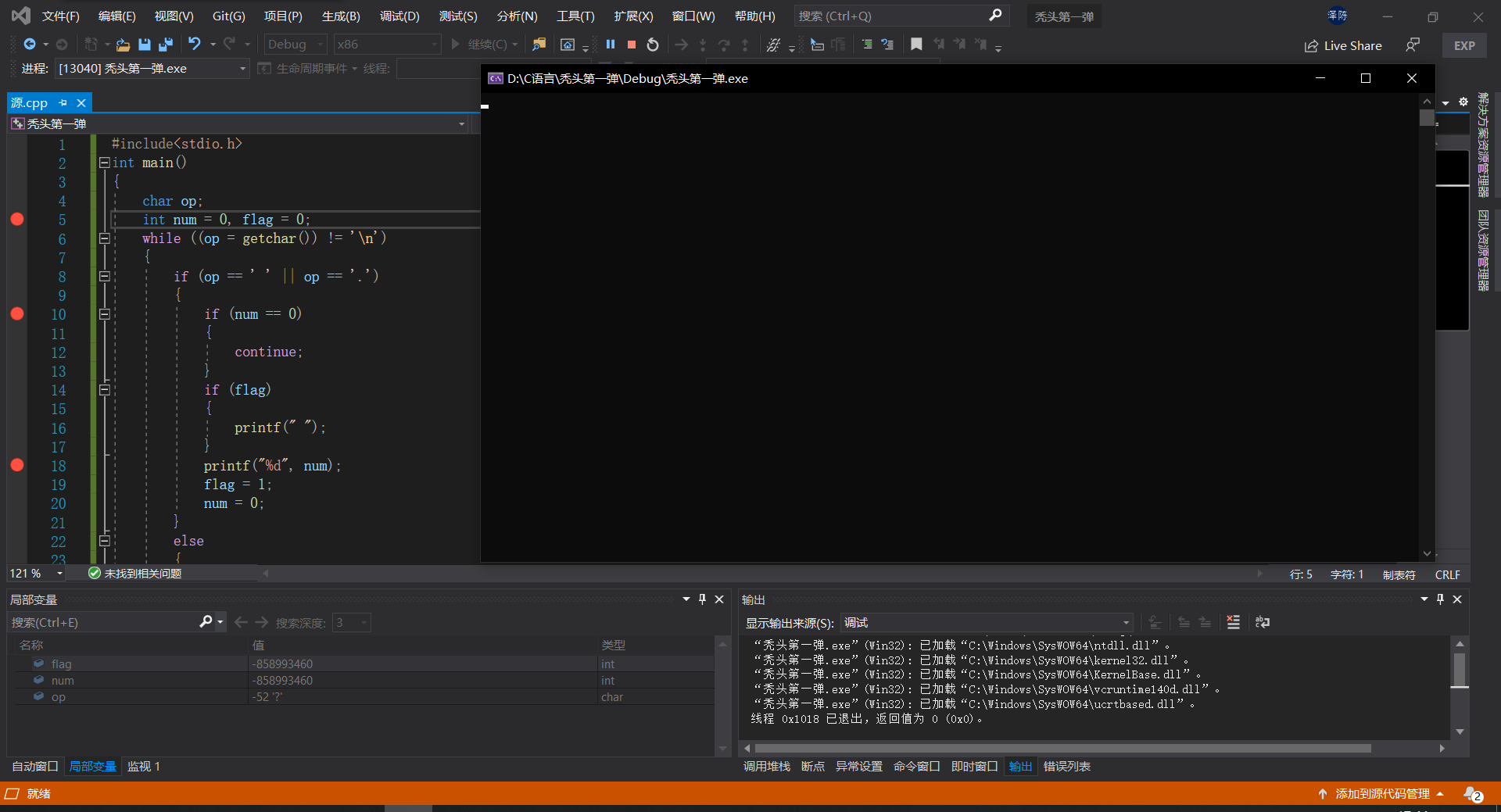### 3.3.2 代码截图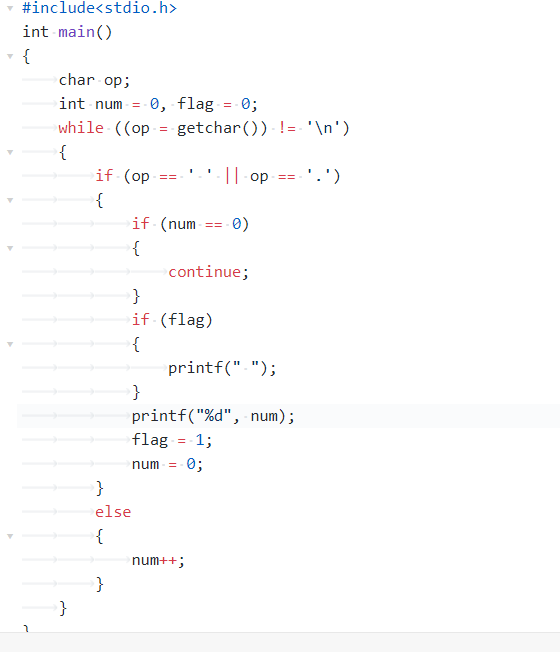### 3.3.3 PTA提交列表及说明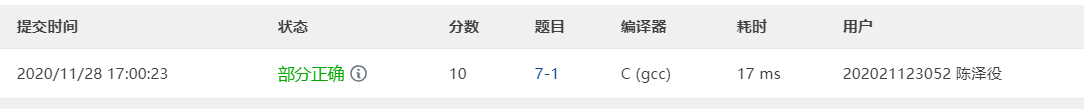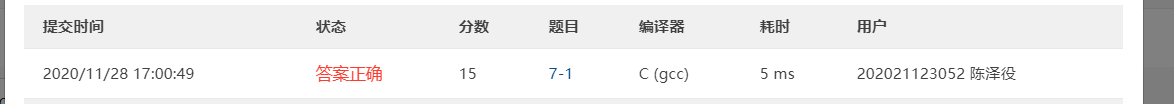• 忽略开头多空格和空句子的原因。
posted @ 2020-11-22 22:57  头秃了没  阅读(48)  评论(0编辑  收藏